# AQA A Level Maths: Pure复习笔记6.1.2 Logarithmic Functions

### Logarithmic Functions

#### Logarithmic functions• a = bx and log b a = x are equivalent statements
• a > 0
• b is called the base
• Every time you write a logarithm statement say to yourself what it means
• log3 81 = 4“the power you raise 3 to, to get 81, is 4”
• logp q = r“the power you raise p to, to get q, is r”

#### Logarithm rules

• A logarithm is the inverse of raising to a power so we can use rules to simplify logarithmic functions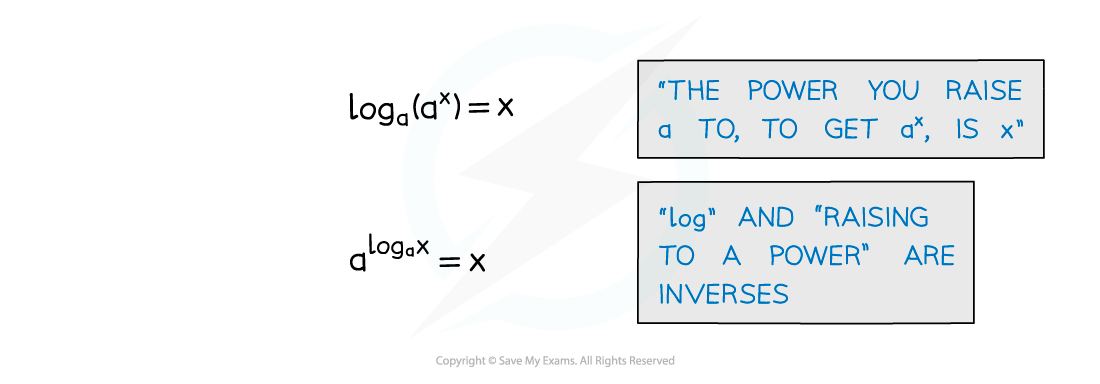• There are more laws of logarithms but these are the only ones needed for A level

#### How do I use logarithms?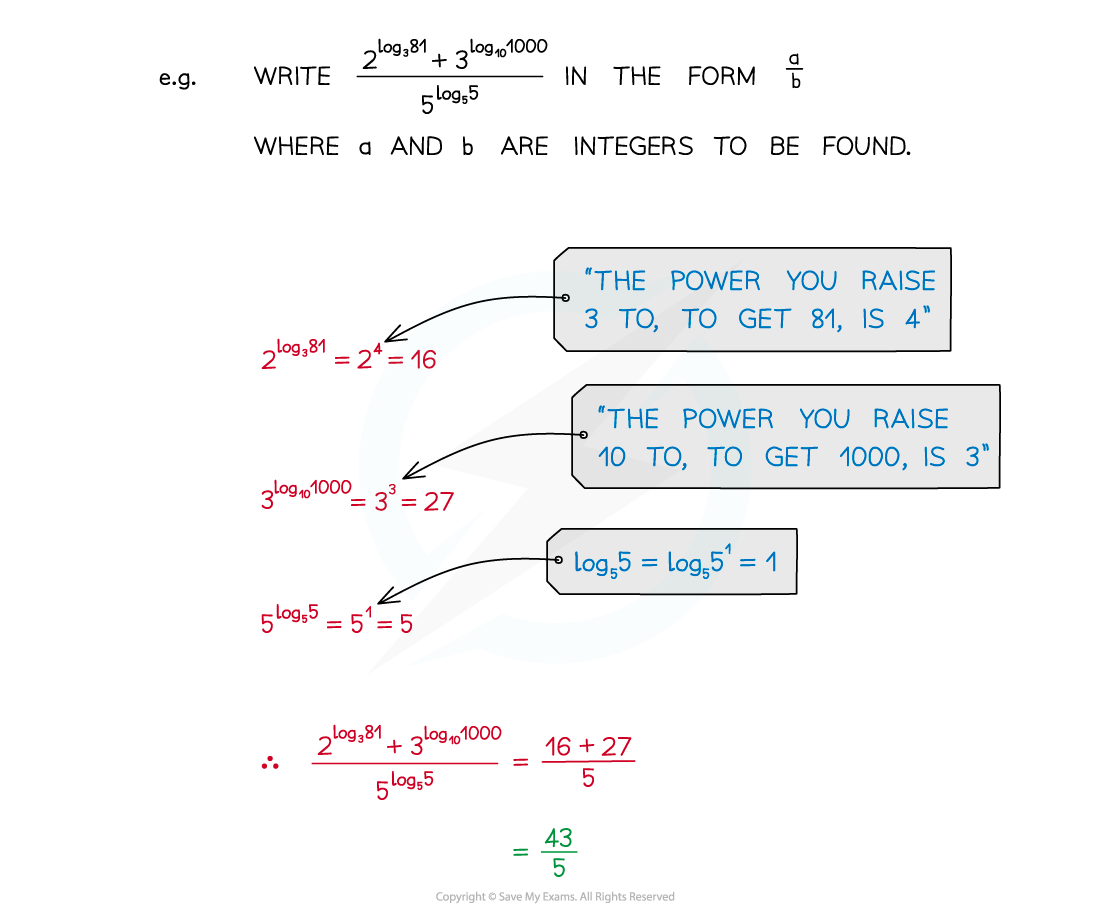•  Recognising the rules of logarithms allows expressions to be simplified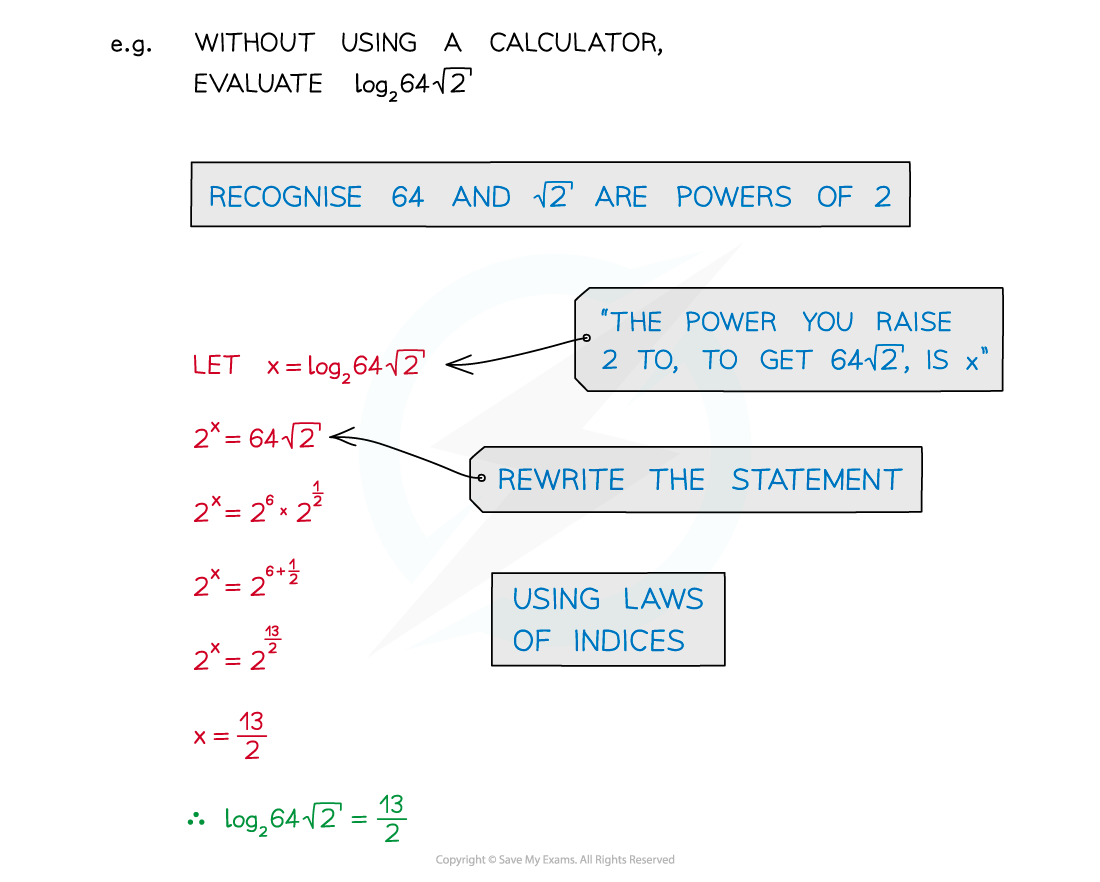• Recognition of common powers helps in simple cases
• Powers of 2: 20 = 1, 21 = 2, 22 = 4, 23 = 8, 24 =16, …
• Powers of 3: 30 = 1, 31 = 3, 32 = 9, 33 = 27, 34 = 81, …
• The first few powers of 4, 5 and 10 should also be familiar

For more awkward cases a calculator is needed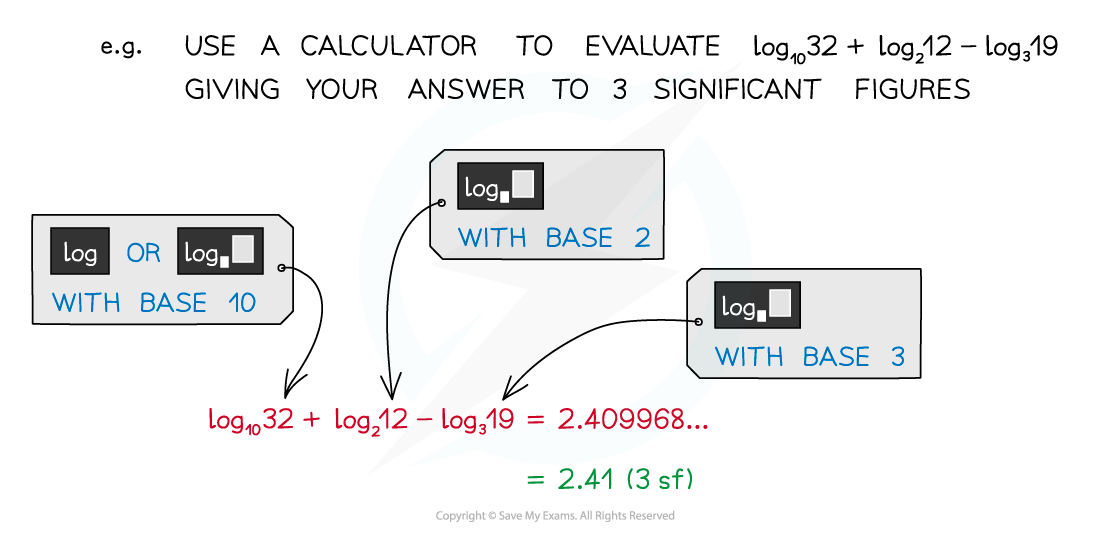• Calculators can have, possibly, three different logarithm buttons• This button allows you to type in any number for the base• Natural logarithms (see “e”)• Shortcut for base 10 although SHIFT button needed
• Before calculators, logarithmic values had to be looked up in printed tables

#### Notation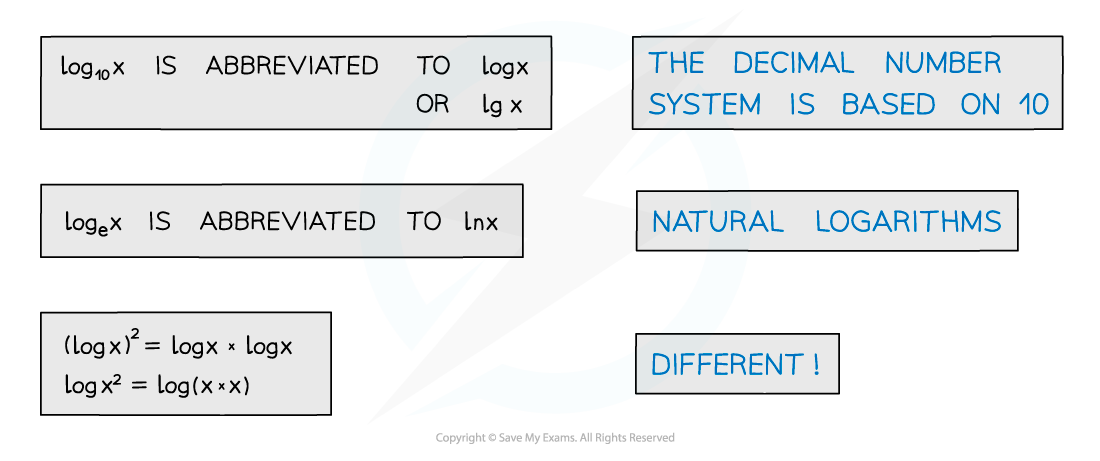• 10 is a common base
• log10 x is abbreviated to log x or lg x
• The value e is another common base
• loge x is abbreviated to ln x
• (log x)2 ≠ log x2

#### Worked Example